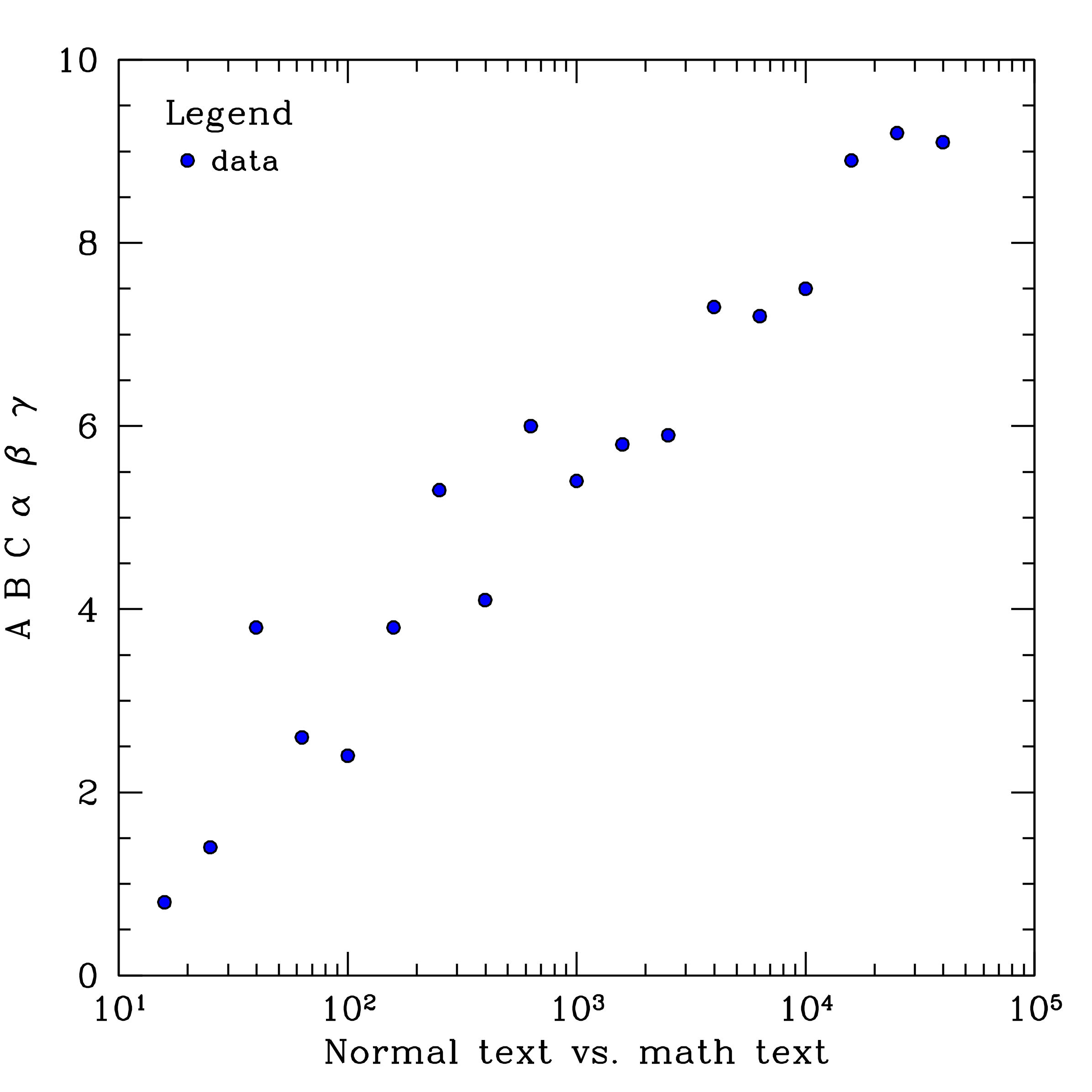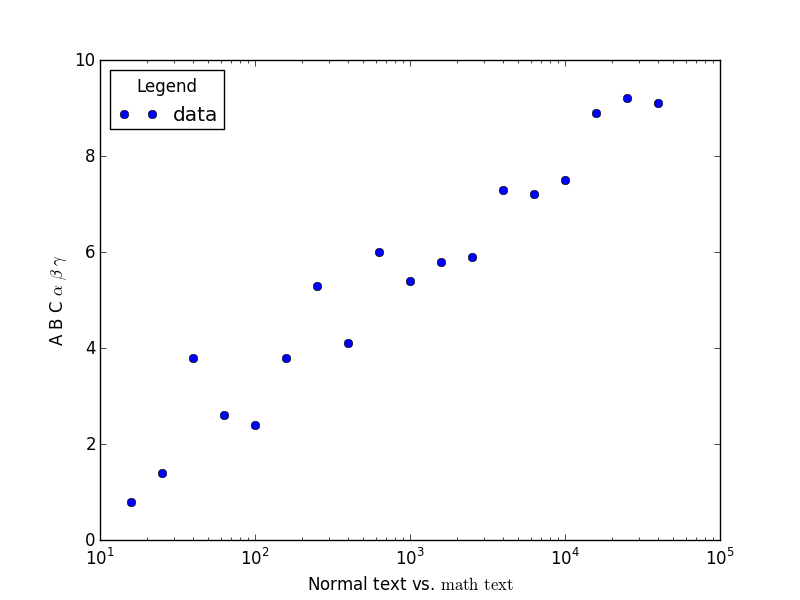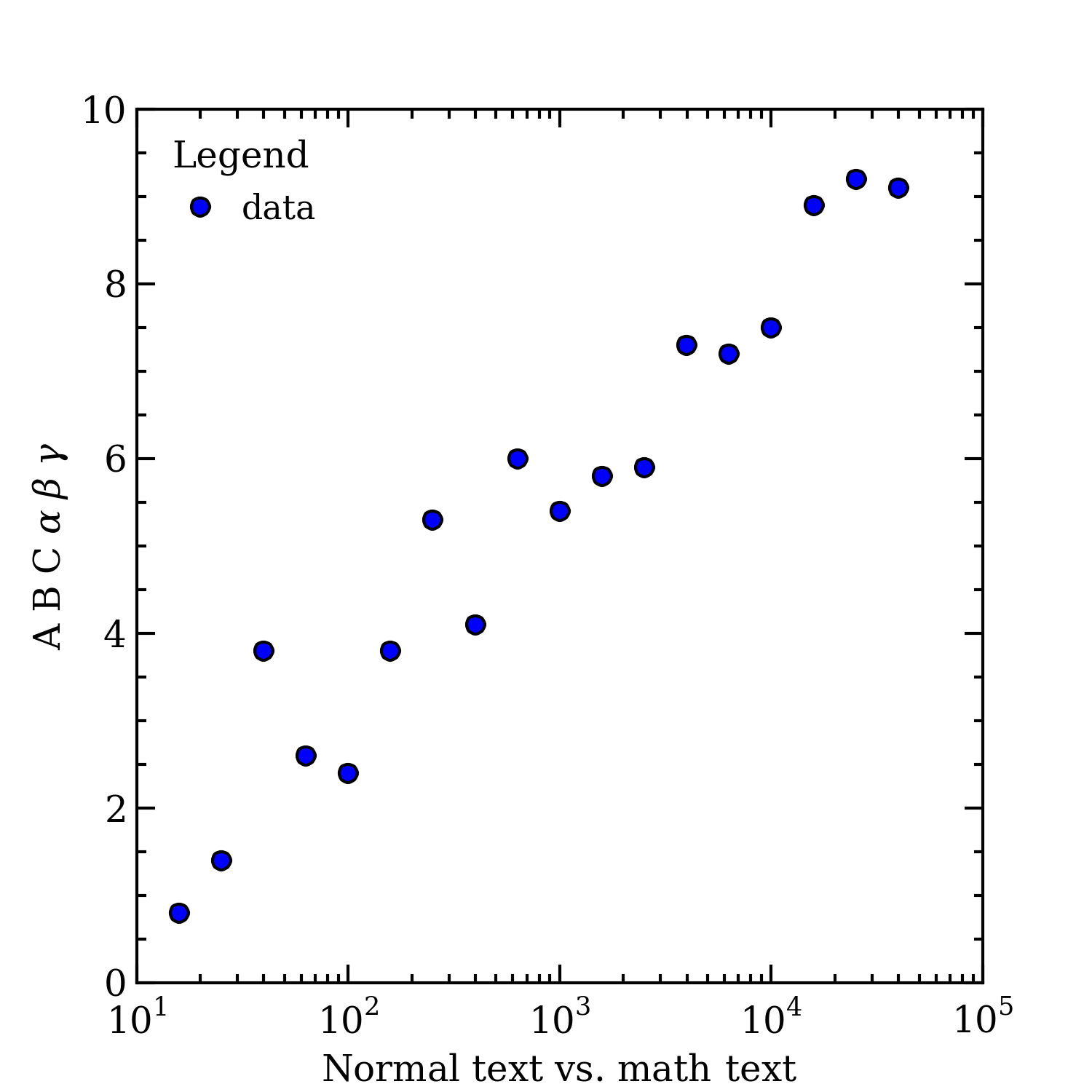## How to make publication-quality Matplotlib plots

This guide is intended for Matplotlib<2.0; if you are using a newer version, please go here.

As someone who likes to use Python and Matplotlib, I have been struggling to make my plots look as nice as those created using Supermongo (SM) or IDL.

Here is a very basic SM macro that I wrote to plot this data, and the resulting figure:And here is the same figure made using this python script and matplotlibrc defaults:Yikes! There are a number of problems with this figure, including: the text size is too small compared to the other elements, there are no minor tickmarks on the y-axis, the tickmarks are too small, and the fonts are mixed.

Luckily, this can be easily fixed. Almost everything that I found needed adjustment can be changed from one's matplotlibrc file. This is what mine looks like:


# Set the backend, otherwise the figure won't show up. Note that this will
# depend on your system setup; to see which backend is the default,
# run "matplotlib.get_backend()" in the Python interpreter.
backend                 :  GTK3Agg

# Increase the default DPI, and change the file type from png to pdf
savefig.dpi             :   300
savefig.extension       :   pdf

# Simplify paths by removing "invisible" points, useful for reducing
# file size when plotting a large number of points
path.simplify           :   True

# Instead of individually increasing font sizes, point sizes, and line
# thicknesses, I found it easier to just decrease the figure size so
# that the line weights of various components still agree
figure.figsize          :   4,4

# In this example I am *not* setting "text.usetex : True", therefore the
# following ensures that the fonts in math mode agree with the regular ones.
#
font.family             :   serif
mathtext.fontset        :   custom

# Increase the tick-mark lengths (defaults are 4 and 2)
xtick.major.size        :   6
ytick.major.size        :   6
xtick.minor.size        :   3
ytick.minor.size        :   3

# Increase the tick-mark widths as well as the widths of lines
# used to draw marker edges to be consistent with the other figure
# linewidths (defaults are all 0.5)
xtick.major.width       :   1
ytick.major.width       :   1
xtick.minor.width       :   1
ytick.minor.width       :   1
lines.markeredgewidth   :   1

# Have the legend only plot one point instead of two, turn off the
# frame, and reduce the space between the point and the label
legend.numpoints        :   1
legend.frameon          :   False


The final touch is adding minor tickmarks (this is done automatically for logarithmic axes, but not for linear). This is not possible to set in matplotlibrc, so I just call ax.minorticks_on() on the axis object(s) in the figure.

    import matplotlib.pyplot as plt
import numpy as np

x, y = np.loadtxt("data.txt", skiprows = 1, unpack = True)
x = 10 ** x

fig, ax = plt.subplots()
ax.semilogx(x, y, 'o', label = "data")
ax.legend(loc = 2, title = "Legend")
ax.set_xlabel(r"Normal text vs. ${\rm math\, text}$")
ax.set_ylabel(r"A B C $\alpha$ $\beta$ $\gamma$")

#
# Turn on minor ticks!!
#
ax.minorticks_on()

fig.savefig("plot.pdf")
plt.close(fig)


And here is the result:Definitely an improvement!